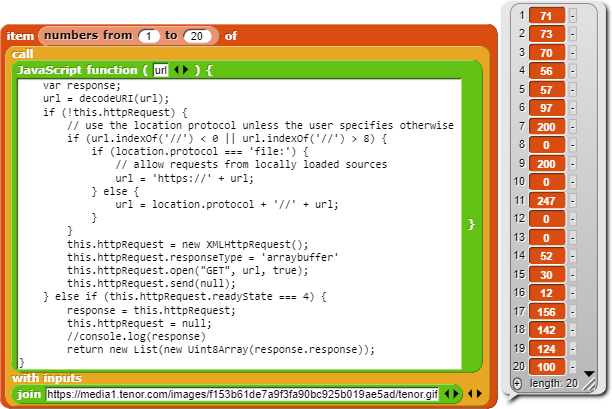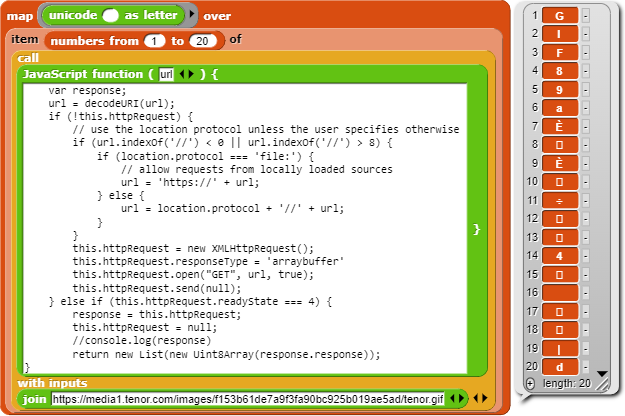# Getting costumes from external sources

the primitive block gives "tainted" costumes,and then the project cannot be saved

YepTried many things last year but never found a way around itTurns out going thru a proxy does work - but it makes us reliant on a proxy and they usually don't last too long or are reliable enough)

Although as I'm typing this, another idea pops into my head .....

CORS(app)

idk if that works

AFAICR Nope

oh ok

A few weeks ago, I made a load binary data from URL reporter (to get at audio data)

It seems to work for images files as wellBut you'll need to code up some data->image decoders - probably not too hard to do if you use JavaScript

Let me know if the technique worksanother simpler way:display the image then screenshot
i found out that screenshots are generally not tainted

How to you do that?

wiill try using proxies after dinner

very much doubt if that approach will work
Turns out I'm wrong on that - going via a proxy does workI found my ancient Snap! .gif decoder project and done a quick bodge to get it to load a gif from a url and that seems to work ok

So you just need to come up with some png and jpeg decoders and your sortedAnd maybe a faster gif one as wellfrom flask import Flask,request
from requests import get
from PIL import Image

CORS(app)
b64 = ""
b64 += "".join(map(chr, range(48, 58)))
b64 += "".join(map(chr, range(65, 91)))
b64 += "".join(map(chr, range(97, 123)))
b64 += "_ "
print(b64)

def b2s(s):
res = ""
image = Image.open(BytesIO(s))
for i in range(image.width):
for j in range(image.height):
r, g, b, a = pixels[i, j]
t = r * 16777216 + g * 65536 + b * 256 + a
res += b64[t & 63] + b64[(t >> 6) & 63]
res += b64[(t >> 12) & 63] + b64[(t >> 18) & 63]
res += b64[(t >> 24) & 63] + b64[(t >> 30) & 63]
res += b64[(t >> 36) & 63]
return res

@app.route('/pixels')
def abc():
return b2s(get(request.args["q"]).content)

@app.route('/proxy')
def proxy():
return get(request.args["q"]).content

app.run(host='0.0.0.0', port=8080)


assumes that you knows the width and height though

I'm not familiar with that sort of JavaScript

oops thats python
the /proxy doesnt work

(yeah im using 18001767679s serverside,he's an expert on flask)
does the /pixels work?(ill have to do some bit manipulation)

let not get snaily and just use ascii

pretty sure the pixels are all there but dunno why is the image transform incorrect
maybe ill send width and height from server after all

from flask import Flask,request
from requests import get
from PIL import Image
from io import BytesIO

CORS(app)
b64 = ""
b64 += "".join(map(chr, range(48, 58)))
b64 += "".join(map(chr, range(65, 91)))
b64 += "".join(map(chr, range(97, 123)))
b64 += "_ "
print(b64)

def b2s(s):
res = ""
image = Image.open(BytesIO(s))
for j in range(image.height):
for i in range(image.width):
r, g, b, a = pixels[i, j]
res+=chr(r)+chr(g)+chr(b)+chr(a)
#t = r * 16777216 + g * 65536 + b * 256 + a
#res += b64[t & 63] + b64[(t >> 6) & 63]
#res += b64[(t >> 12) & 63] + b64[(t >> 18) & 63]
#res += b64[(t >> 24) & 63] + b64[(t >> 30) & 63]
#res += b64[(t >> 36) & 63]
return res
def dims(s):
image = Image.open(BytesIO(s))
return str(image.width)+","+str(image.height)

@app.route('/pixels')
def abc():
return b2s(get(request.args["q"]).content)

@app.route('/dims')
def dim():
return dims(get(request.args["q"]).content)

app.run(host='0.0.0.0', port=8080)